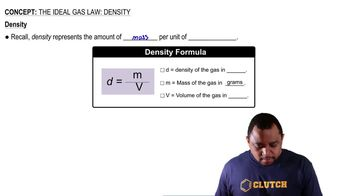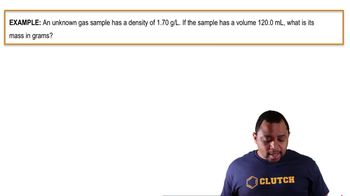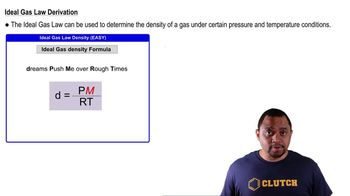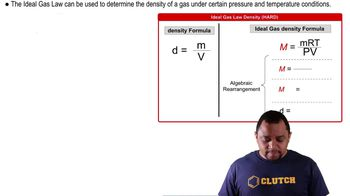Start typing, then use the up and down arrows to select an option from the list.# The Ideal Gas Law: Density

Jules Bruno
193views
So if you clicked on this video, that means you want to see how we derive this new version of the ideal gas law. So it all begins with our density formula. Remember, density equals M over V. Remembering this, we move on to the ideal gas law where we're dealing with Mueller Mass. Remember, Moeller Mass equals Marty over PV, and we just said that M over V represents density. So take them out and put in density. We still have our tea and overpay. We need to isolate density. So just use algebra to isolate it. Multiply both sides by pressure. So Moeller mass times pressure equals d rt. Isolate our density by dividing up Artie and we see now that density equals p times em over r t So that's how we went about an isolated and derived this new formula for density. Now this is how we derived it. But just remember, dreams push me over rough times, knowing that helps you to write the formula very quickly. Now that we've seen this formula, click on to the next video and let's put it to work00:4301:2101:0301:1902:42